# Cell References in Excel

## Introduction

There are two types of cell references: relative and absolute. Relative and absolute references behave differently when copied and filled to other cells. Relative references change when a formula is copied to another cell. Absolute references, on the other hand, remain constant, no matter where they are copied.

## Relative Cell References

The default cell references are relative references. See the picture below.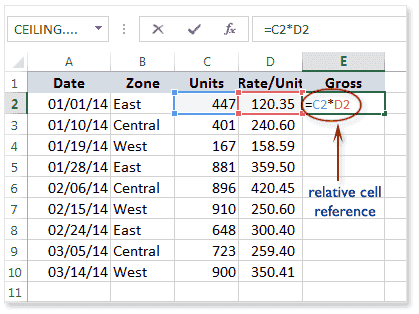When copied across multiple cells, they change based on the relative position of rows and columns. For example, if you copy the formula =C2*D2 from row 2 to row 3, the formula will become =C3*D3. Relative references are especially convenient whenever you need to repeat the same calculation across multiple rows or columns.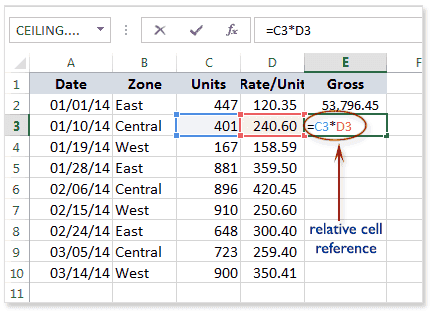Press Enter key on the keyboard. The formula will be calculated, and the result will be displayed in the cell.

To create and copy a formula using relative references:

In the following example, we want to create a formula that will calculate the gross by multiplying the units with rate/unit. It is better to create a formula and copy the formula for each row rather than to create a formula for each row. Here in the example below we have written the formula in cell E2 and drag it below or double click the autofill option or copy it to the other rows. The cells will be relatively changed.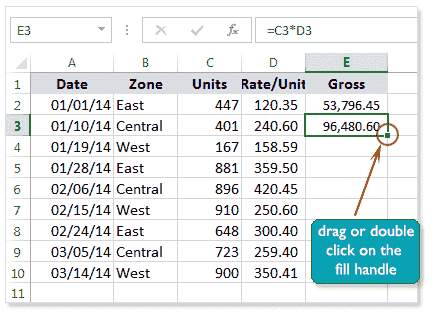Here is the picture below after copying the formula for each of the rows.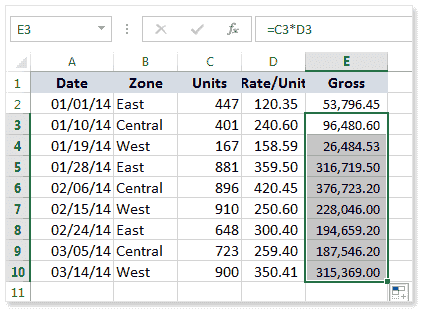Here in the picture below shows the formula in cell E7 is referencing the row 7, i.e. C7 * D7.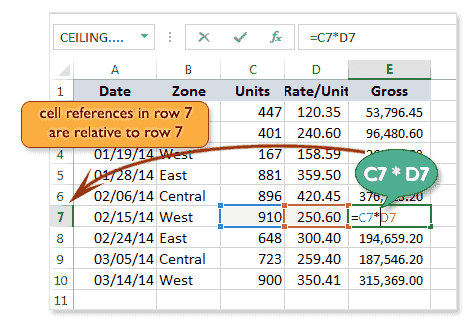## Absolute Cell Reference

Sometimes we need to copy a formula that, the content of some cell associated with this formulas must be fixed. In that condition, the relative cell references can be used. In this type of cell references, we can keep the row and/or column constant.

An absolute reference is designated in a formula by the addition of a dollar sign (\$). It can precede the column reference or the row reference or both.

The table below shows that the usage of absolute cell reference.

 Absolute Reference Particular Keys in the keyboard \$A\$1 The column and the row do not change when copied. Press F4. A\$1 The row does not change when copied. Press F4 twice . \$A1 The column does not change when copied. Press F4 three times .

You will generally use the \$A\$1 format when creating formulas that contain absolute references. The other two formats are used much less frequently.

When writing a formula, you can press the F4 key on your keyboard to switch between relative and absolute cell references. This is an easy way to quickly insert an absolute reference.

Create and copy a formula using absolute references:

If we want to calculate the commission for each row by 5% of gross, we have to use the absolute cell reference. By default the cell reference is relative and it makes changes the cell address at the time of copying the formula.

Here in the example below, we have written the formula in the cell F5. Here we see that the E5 is multiplying with \$D\$1, that means that every value of column E will be multiplied by the value of column D and row 1. The \$ (dollar) sign have restricted to change the cell address. Press enter key to the cell F5 to see the result or to stay on that press Ctrl+enter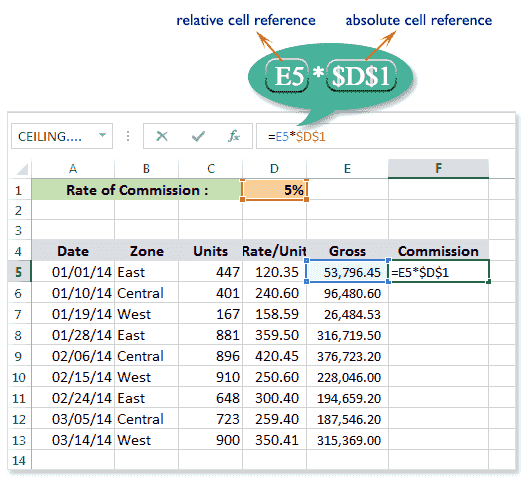Now locate the fill handle in the cell where the formula has been written and press and hold the mouse key on the fill handle then drag upto the cell you desire to copy and release the mouse button. You can also double-click on the fill handle to copy the formula upto the cell automatically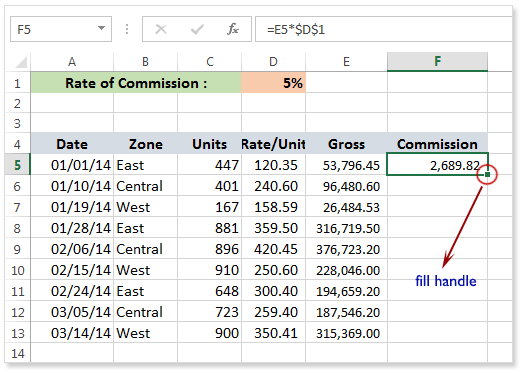Here is the picture below shows, after copies the formula for a number of rows.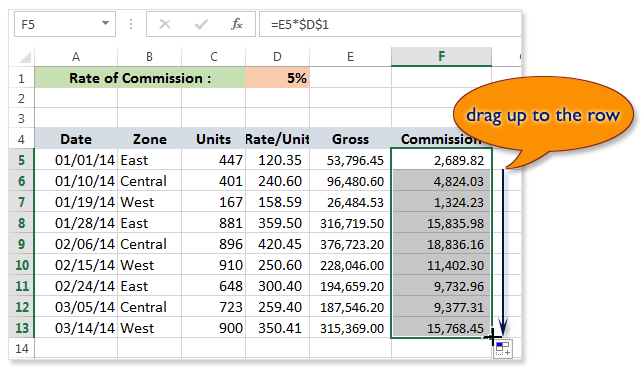Now you see, how the absolute cell reference works.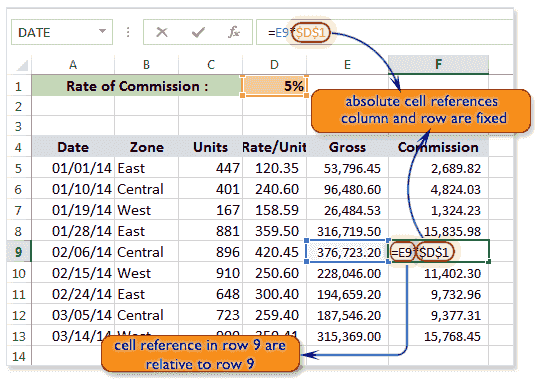## Mixed Reference

Sometimes we need such a combination of formulas that contain such a cell references the can be static for the rows or columns, i.e. a combination of relative and absolute references (mixed reference).

The below sheet shows that a company has set a target for the 1st. Qtr. for two product TV (LCD) and TV (LED) and also specified the achievable target for the months of the Qtr. and calculate the units to be achieved for the 3 months. Suppose the target is 75000 and 12000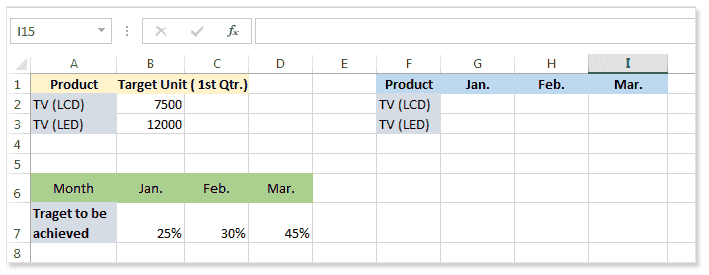See the following example, we want to get the number of units to be produced for January to get the setting target. Here in the sheet according to the condition we have multiplied B2 by B7.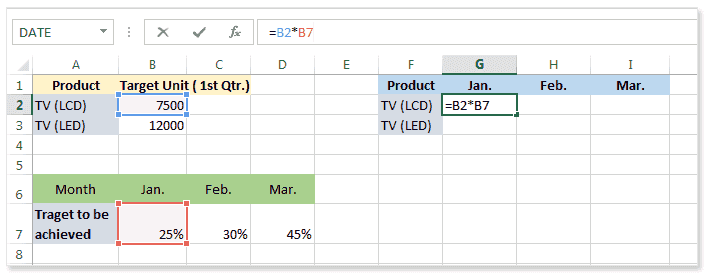And now, we need to copy the formula for the month of February, and here we see the cell references are relative and the result is incorrect. See the picture below.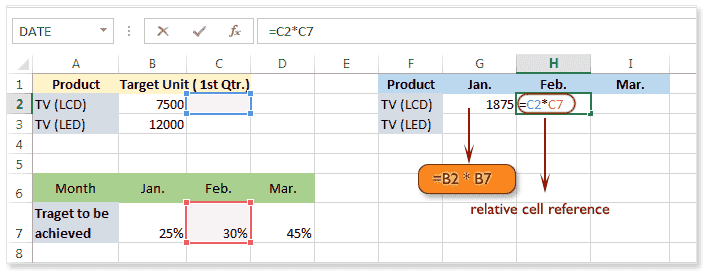To prevent this situation we have to use the mixed cell references. We have used \$B2, that means if we copy the formula horizontally or vertically the column will be absolute and row will be relative. In the same way, we have used C\$7, that means if we copy the formula horizontally or vertically the column will be relative but row will be static. Here is the picture below.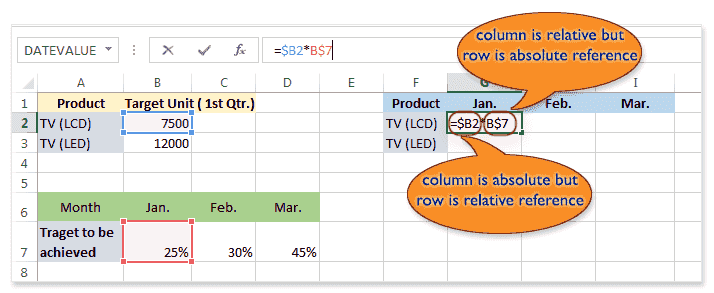See the picture below for TV (LCD) for the month February.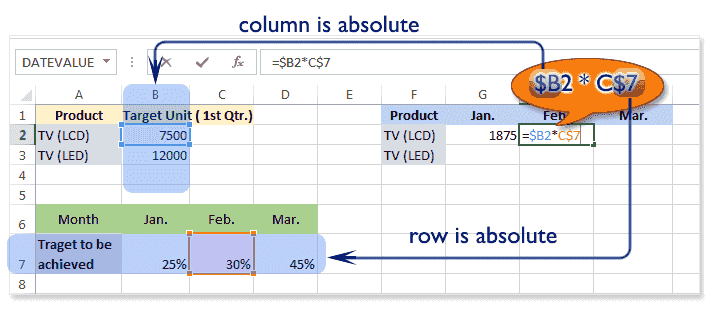See the picture below for TV (LED) for the month of January.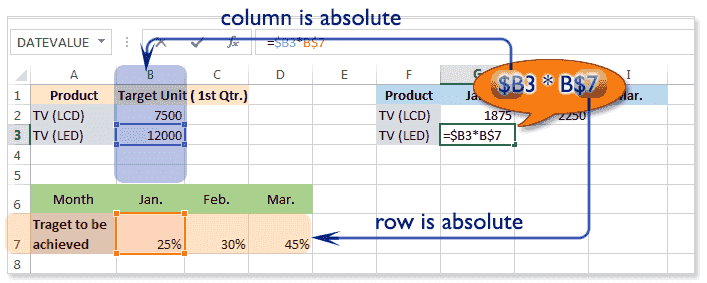How using cell references with multiple worksheets ?

Excel allows cell references not only within one sheet of a workbook but also can update many sheets at a time with the changes of value of one cell of a sheet. To work with more sheets, the cell address denotes like the picture below.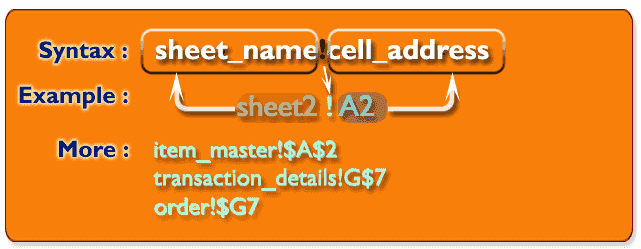Here is the picture below shows the active sheet master and another inactive sheet transaction. We want to write the formula in transaction sheet with the usage of cell reference of master sheet.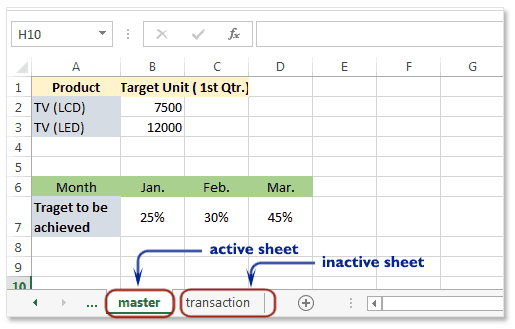Here is the picture below. Here in the formula [master!\$B2] indicating that, the sheet is 'master' and the cell address is \$B2, that is in the sheet 'master' the column B is absolute and row is relative. In the formula [master!B\$7] indicating that, the sheet is 'master' and the cell address is B\$7, that is in the sheet 'master' the column is relative and row7 is absolute. In the formula [transaction!\$B2] indicating that, the sheet is 'transaction' and the cell address is \$B2, that is in the sheet 'transaction' the column B is absolute and row is relative.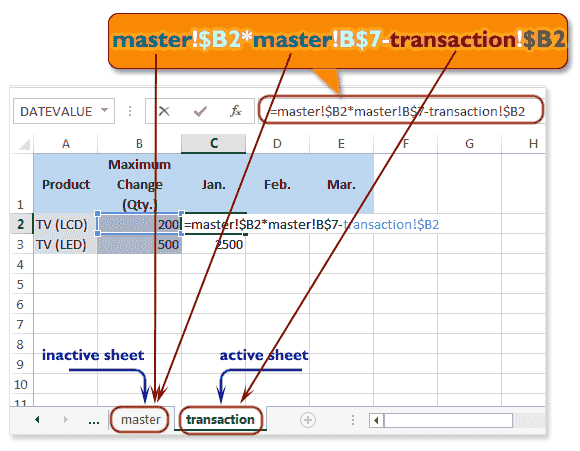Note that if a worksheet name contains a space, you will need to include single quotation marks (' ') around the name. For example 'Cell Reference'!|\$F\$2.

Previous: Basics of Cell - Excel 2013
Next: Functions Basic - Excel 2013

﻿